# An introduction to the Registration ProblemFigure 1: Pinch Force measured over time seconds for subjects . The blue curve shows the mean .

To explain the registration problem i will start with an example. In Figure 1 the pinch force dataset is shown, to collect the data a group of 20 subjects were asked to press a button as hard if they can after they hear a sound signal. The pressure was then recorded every 2 milliseconds, resulting in 151 observations. Since reaction times of the subjects differ we can clearly see some shift in the curves reflecting the pressure. The problem with this kind of shifted data is now that even very simple statistical measures, like mean or variance, are not meaningful. To see this take a look at the blue curve which is the sample mean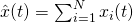. It is visible, that the mean curve does not reflect the shape of the sample curves and even worse the highest point of this curve is smaller than the smallest sample curve. To obtain information about the mean pressure this curve is therefore a bad measure. In case of the pinch fore data an obvious way to fix this problem is to align the curves at certain landmarks, for example the peaks of each curve. This is landmark registration is for example covered by [1, 2],  or . However, Landmark registration has certain drawbacks. Considering more complex problems defining the Landmarks becomes ugly very fast, especially when working with more than one spatial dimension. It is also not clear how to choose the Landmarks, consider for example curves where some are wider than others, in this case sometimes also inflection points are used as landmarks. This then leads to a methods which rely on minimizing a distancebetween registered functionsand a template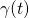, for example one curve out of the sample. See , , , or  for more insights. This strategy works very well in many situations but also has severe problems. Consider for example a sample of curves where some curve have one peak while others have two. A registration method that minimizing a distance between the curves and a one peaked template, will then then to pinch the curves with two peaks, see .Figure 2: Registered curves using landmark registration. The structural mean now reflects the characteristics of the sample curves

An alternative approach was developed by  and . Where registration was considered as a tool for statistical analysis. Whenever the random functionspossess “bounded shape variation”, then there exists a finiteand warping functionssuch that with probability 1

(1)for some basis functionsand individually different coefficients. An advantage of this way to look at the registration problem that it allows curves to be registered with a more complex structure than the curves displayed in figure 1. Traditional registration procedures can be understood as a registration with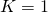and are troubled with curves as displayed in figure 3. Decomposition 1 is unfortunately not unique if. There will then exist different sets of basis functionsandsuch that

(2)The corresponding spacesand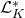spanned byand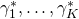, respectively, may be structurally very different from each other. As an example consider continuous periodic functions with period length equal to 1, and assume that in each period every curve just possesses one local maximum and one minimum. Registration is driven by the succession of local extrema (shape features) in each of the functions. For any continuous functionone can determine locationsand heightsof all isolated local extrema in the interior of. This means that for allthere exists an open neighborhoodofsuch that eitherfor allor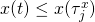for all. Iflet, and letdenote the corresponding-dimensional vector of heights of local extrema (including starting and end points). When analyzing such functionson the interval, periodicity just means that,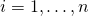. If each of the curves just has one maximum and one minimum, then, and by. It is indeed simple to construct a 3 dimensional spaceanalytically. For example, let,,, and. Quite obviously, for anythere exists a unique elementwithand. We can thus conclude that there are unique warping functionsand unique coefficientssuch that

(3)Note that the functionshave their local extrema at different locations, depending on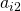and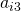. Registration totherefore does not lead to an alignment of shape features. Butis not the only possible candidate space. Consider the spaceof all polynomialsof order 5 satisfying the constraintsas well as. This is again a three dimensional space of functions with identical starting and end points, while thegenerates functions with one local maximum and one minimum in the interior of. There thus exists a set of warping functionssuch that. The two spacesandare not identical. As a matter of fact one can construct arbitrary many candidate spacesby pre-chosen an arbitrary set of basis functionsas long as there exists awith. A central question which spacecan be considered as the “best”.  answered this question by selecting the linear subspace where the least amount of warping is necessary.

### References

 F. L. Bookstein, Morphometric tools for landmark data: geometry and biology, Cambridge University Press, 1997.
[Bibtex]
@book{bookstein1997morphometric,
title={Morphometric Tools for Landmark Data: Geometry and Biology},
author={Bookstein, F.L.},
isbn={9780521585989},
lccn={lc91039063},
series={Geometry and Biology},
year={1997},
publisher={Cambridge University Press}
}
 F. L. Bookstein, The measurement of biological shape and shape change, Springer, 1978.
[Bibtex]
@book{bookstein1998,
AUTHOR = "Bookstein, F.L.",
TITLE = "The Measurement of Biological Shape and Shape Change",
PUBLISHER = "Springer",
YEAR = "1978",
BIBSOURCE = "http://www.visionbib.com/bibliography/describe448.html#TT52072"}A. Kneip and T. Gasser, “Statistical tools to analyze data representing a sample of curves,” The annals of statistics, vol. 20, iss. 3, p. 1266–1305, 1992.
[Bibtex]
@article{kneip1992,
ajournal = "Ann. Statist.",
author = "Kneip, Alois and Gasser, Theo",
doi = "10.1214/aos/1176348769",
journal = "The Annals of Statistics",
month = "09",
number = "3",
pages = "1266--1305",
publisher = "The Institute of Mathematical Statistics",
title = "Statistical Tools to Analyze Data Representing a Sample of Curves",
url = "http://dx.doi.org/10.1214/aos/1176348769",
volume = "20",
year = "1992"
}
 T. Gasser and A. Kneip, “Searching for structure in curve sample,” Journal of the american statistical association, vol. 90, iss. 432, pp. 1179-1188, 1995.
[Bibtex]
@article{gasser:95,
ISSN = {01621459},
URL = {http://www.jstor.org/stable/2291510},
abstract = {The shape of a regression curve can to a large extent be characterized by the succession of structural features like extrema, inflection points, and so on. When analyzing a sample of regression curves, it is often important to know at an early stage of data analysis which structural features are occurring consistently in each curve of the sample. Such a definition is usually not easy due to substantial interindividual variation both in the x and the y axis and due to the influence of noise. A method is proposed for identifying typical features without relying on an a priori specified functional model for the curves. The approach is based on the frequencies of occurrence of structural features, as, for example, maxima in the curve sample along the x axis. Important tools are nonparametric regression and differentiation and kernel density estimation. Apart from a theoretical foundation, the usefulness of the method is documented by application to two interesting biomedical areas: growth and development, and neurophysiology.},
author = {Gasser, Theo and Kneip, Alois },
journal = {Journal of the American Statistical Association},
number = {432},
pages = {1179-1188},
publisher = {Taylor & Francis, Ltd.},
title = {Searching for Structure in Curve Sample},
volume = {90},
year = {1995}
}
 H. Sakoe and S. Chiba, “Dynamic programming algorithm optimization for spoken word recognition,” Acoustics, speech and signal processing, ieee transactions on, vol. 26, iss. 1, p. 43–49, 1978.
[Bibtex]
@article{sakoe1978,
abstract = {This paper reports on an optimum dynamic progxamming (DP) based time-normalization algorithm for spoken word recognition. First, a general principle of time-normalization is given using time-warping function. Then, two time-normalized distance definitions, called symmetric and asymmetric forms, are derived from the principle. These two forms are compared with each other through theoretical discussions and experimental studies. The symmetric form algorithm superiority is established. A new technique, called slope constraint, is successfully introduced, in which the warping function slope is restricted so as to improve discrimination between words in different categories. The effective slope constraint characteristic is qualitatively analyzed, and the optimum slope constraint condition is determined through experiments. The optimized algorithm is then extensively subjected to experimental comparison with various DP-algorithms, previously applied to spoken word recognition by different research groups. The experiment shows that the present algorithm gives no more than about two-thirds errors, even compared to the best conventional algorithm.},
author = {Sakoe, H. and Chiba, S. },
booktitle = {Acoustics, Speech and Signal Processing, IEEE Transactions on},
citeulike-article-id = {3496861},
journal = {Acoustics, Speech and Signal Processing, IEEE Transactions on},
keywords = {dtw, litreview, thesis},
number = {1},
pages = {43--49},
posted-at = {2008-11-08 22:11:03},
priority = {0},
title = {Dynamic programming algorithm optimization for spoken word recognition},
url = {http://ieeexplore.ieee.org/xpls/abs\_all.jsp?arnumber=1163055},
volume = {26},
year = {1978}
}J. O. Ramsay and X. Li, “Curve registration,” Journal of the royal statistical society: series b (statistical methodology), vol. 60, iss. 2, p. 351–363, 1998.
[Bibtex]
@article {Ramsay19982,
author = {Ramsay, J. O. and Li, Xiaochun},
title = {Curve registration},
journal = {Journal of the Royal Statistical Society: Series B (Statistical Methodology)},
volume = {60},
number = {2},
publisher = {Blackwell Publishers Ltd.},
issn = {1467-9868},
url = {http://dx.doi.org/10.1111/1467-9868.00129},
doi = {10.1111/1467-9868.00129},
pages = {351--363},
keywords = {Dynamic time warping, Geometric Brownian motion, Monotone functions, Spline, Stochastic time, Time warping},
year = {1998},
}J. O. Ramsay, “Estimating smooth monotone functions,” Journal of the royal statistical society: series b (statistical methodology), vol. 60, iss. 2, p. 365–375, 1998.
[Bibtex]
@article {Ramsay1998,
author = {Ramsay, J. O.},
title = {Estimating smooth monotone functions},
journal = {Journal of the Royal Statistical Society: Series B (Statistical Methodology)},
volume = {60},
number = {2},
publisher = {Blackwell Publishers Ltd.},
issn = {1467-9868},
url = {http://dx.doi.org/10.1111/1467-9868.00130},
doi = {10.1111/1467-9868.00130},
pages = {365--375},
keywords = {Convex functions, Density estimation, Generalized additive model, Linear differential equation, Monotonicity, Nonparametric regression, Regression spline, Spline smoothing},
year = {1998},
}A. Kneip, X. Li, K. B. MacGibbon, and J. O. Ramsay, “Curve registration by local regression,” Canadian journal of statistics, vol. 28, iss. 1, p. 19–29, 2000.
[Bibtex]
@article{KneipGib2000,
abstract = {{Functional data analysis involves the extension of familiar statistical procedures such as principal-components analysis, linear modelling and canonical correlation analysis to data where the raw observation is a function x, (t). An essential preliminary to a functional data analysis is often the registration or alignment of salient curve features by suitable monotone transformations hi(t). In effect, this conceptualizes variation among functions as being composed of two aspects: phase and amplitude. Registration aims to remove phase variation as a preliminary to statistical analyses of amplitude variation. A local nonlinear regression technique is described for identifying the smooth monotone transformations hi, and is illustrated by analyses of simulated and actual data.}},
address = {Facult\'{e} des sciences \'{e}conomiqu.es, sociales etpolitiques Universit\'{e} catholique de Louvain, Place Montesquieu 4 B-1348 Louvain-la-Neuve, Belgium; no e-mail address available 700 North Alabama Street, Indianapolis, IN 46204, USA; D\'{e}p. de math\'{e}matiques, Universit\'{e} du Qu\'{e}bec \{a} Montr\'{e}al C. P. 8888 Succursale centre-ville, Montr\'{e}al (Quebec), Canada H3C 3P8; Dept. of Psychology, McGill University 1205 avenue Docteur-Penfield, Montreal (Quebec), Canada H3A 1B1},
author = {Kneip, A. and Li, X. and MacGibbon, K. B. and Ramsay, J. O.},
citeulike-article-id = {6101184},
doi = {10.2307/3315251.n},
issn = {1708-945X},
journal = {Canadian Journal of Statistics},
keywords = {alignment},
number = {1},
pages = {19--29},
posted-at = {2009-11-12 12:34:54},
priority = {2},
title = {{Curve registration by local regression}},
url = {http://dx.doi.org/10.2307/3315251.n},
volume = {28},
year = {2000}
}
 A. Kneip and J. O. Ramsay, “Combining registration and fitting for functional models,” Journal of the american statistical association, vol. 103, iss. 483, pp. 1155-1165, 2008.
[Bibtex]
@ARTICLE{Kneip2008,
title = {Combining Registration and Fitting for Functional Models},
author = {Kneip, Alois and Ramsay, James O},
year = {2008},
journal = {Journal of the American Statistical Association},
volume = {103},
number = {483},
pages = {1155-1165},
url = {http://EconPapers.repec.org/RePEc:bes:jnlasa:v:103:i:483:y:2008:p:1155-1165}
}H. Wagner and A. Kneip, “Nonparametric registration to low-dimensional function spaces,” Computational statistics & data analysis, 2019.
[Bibtex]
@article{WAGNER2019,
title = "Nonparametric registration to low-dimensional function spaces",
journal = "Computational Statistics & Data Analysis",
year = "2019",
issn = "0167-9473",
doi = "https://doi.org/10.1016/j.csda.2019.03.004",
url = "http://www.sciencedirect.com/science/article/pii/S0167947319300714",
author = "Heiko Wagner and Alois Kneip",
keywords = "Amplitude variation, Genes, Dimension reduction, Functional data analysis, Functional principal components, Low dimensional linear function spaces, Phase variation, Registration, Time warping",
abstract = "Registration aims to decompose amplitude and phase variation of samples of curves. Phase variation is captured by warping functions which monotonically transform the domains. Resulting registered curves should then only exhibit amplitude variation. Most existing methods assume that all sample functions exhibit a typical sequence of shape features like peaks or valleys, and registration focuses on aligning these features. A more general perspective is adopted which goes beyond feature alignment. A registration method is introduced where warping functions are defined in such a way that the resulting registered curves span a low dimensional linear function space. The approach may be used as a tool for analyzing any type of functional data satisfying a structural regularity condition called bounded shape variation. Problems of identifiability are discussed in detail, and connections to established registration procedures are analyzed. The method is applied to real and simulated data."
}`

This site uses Akismet to reduce spam. Learn how your comment data is processed.